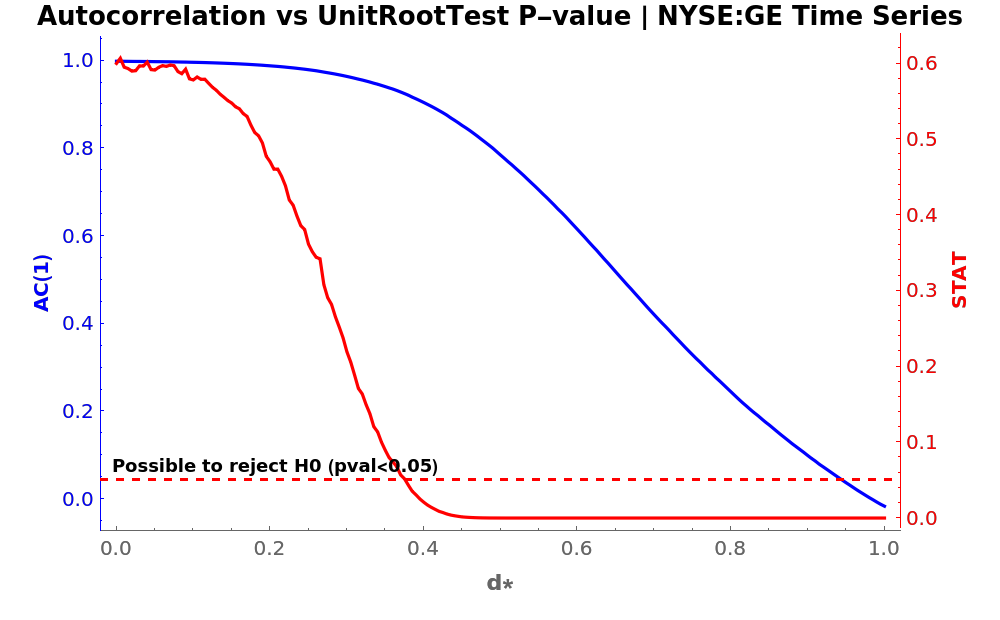Function Repository Resource:

# FractionalDTimeSeries

Calculate the fractional difference of a time series

Contributed by: Frank Salvador Ygnacio Rosas
 ResourceFunction["FractionalDTimeSeries"][tseries,d] applies a fractional difference of order d to the time series tseries. ResourceFunction["FractionalDTimeSeries"][tseries,d,tol] uses a tolerance value of tol.

## Details

ResourceFunction["FractionalDTimeSeries"][tseries,d,tol] represents a fractional difference operator of order d for the time series tseries, given a tolerance tol, as formulated by Marcos Lopez de Prado (2018).
The order d should be a positive real.
By default, the tolerance is set to 0.0001.

## Examples

### Basic Examples (2)

Get a time series of closing prices and visualize it:

 In:=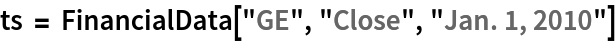Out=In:=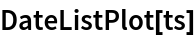Out=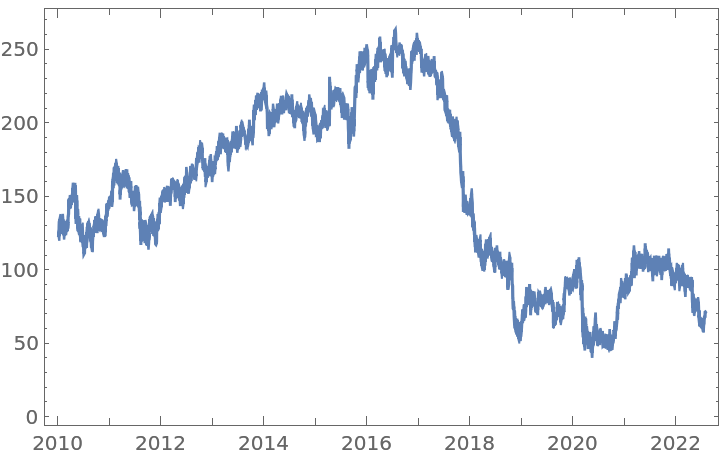Compute the half difference of the time series and visualize it:

 In:=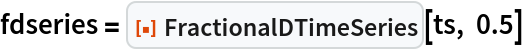Out=In:=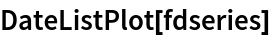Out=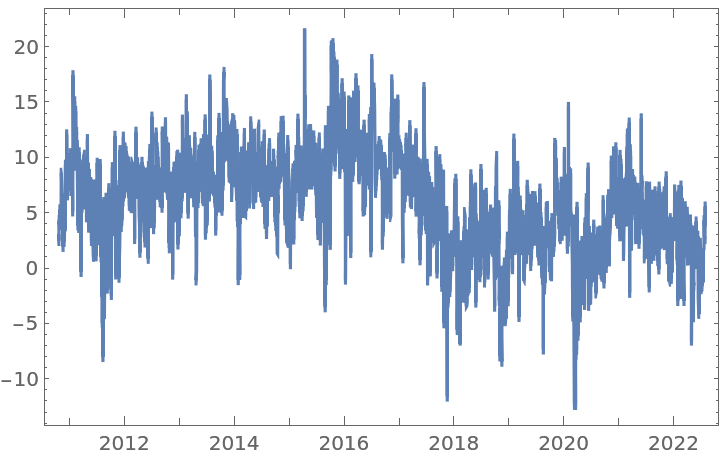### Scope (2)

Get a time series of closing prices:

 In:=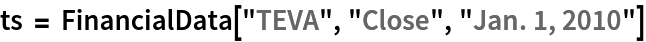Out=In:=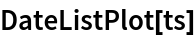Out=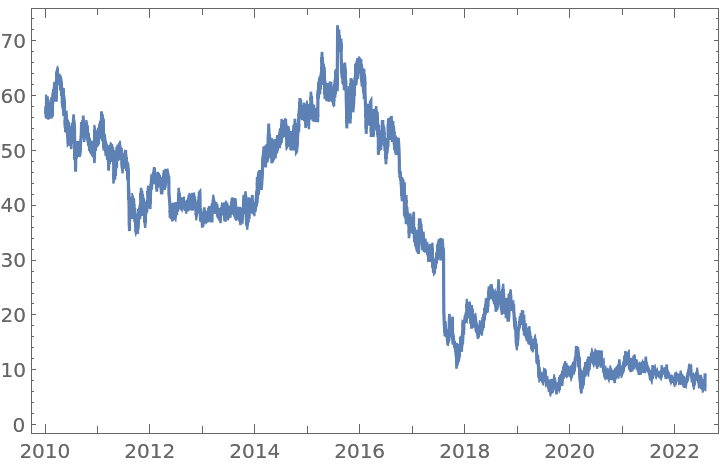With order 1, FractionalDTimeSeries gives the usual first difference:

 In:=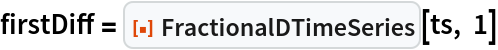Out=In:=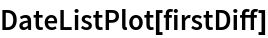Out=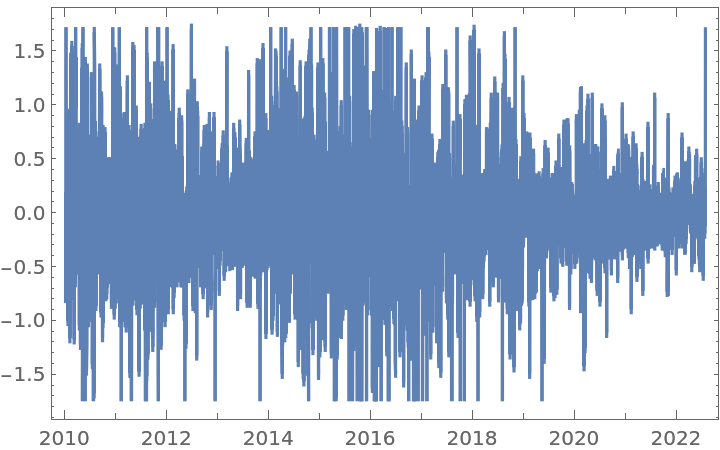However, applying the half-difference twice on the original time series yields an equivalent first difference, but with fewer data points and on a slightly different scale:

 In:=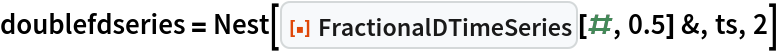Out=In:=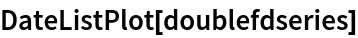Out=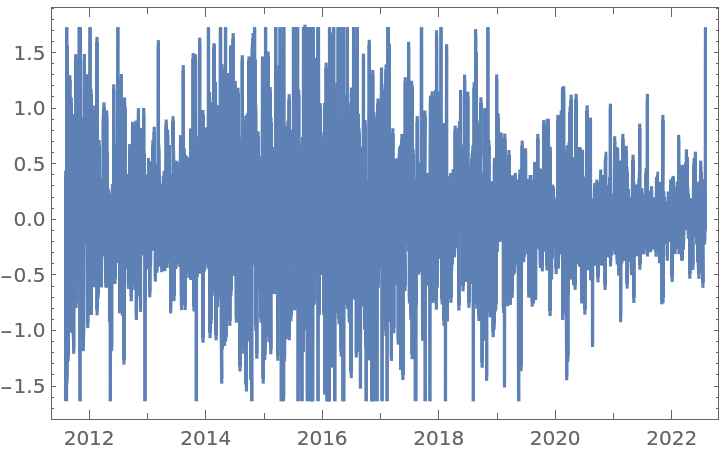Get a time series of closing prices:

 In:=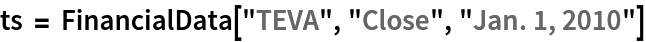Out=Apply a fractional difference of order 0.3531 to the original time series with the default tolerance:

 In:=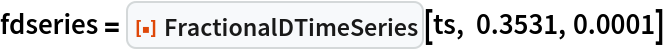Out=In:=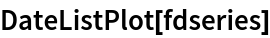Out=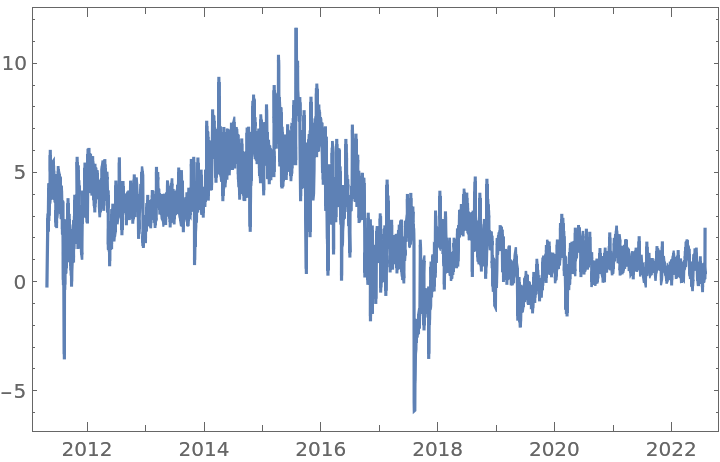For the same time series, a fractional difference of order 0.3531 and a larger tolerance will generate a time series with more data points:

 In:=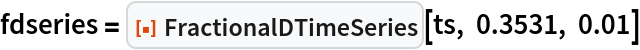Out=In:=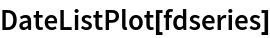Out=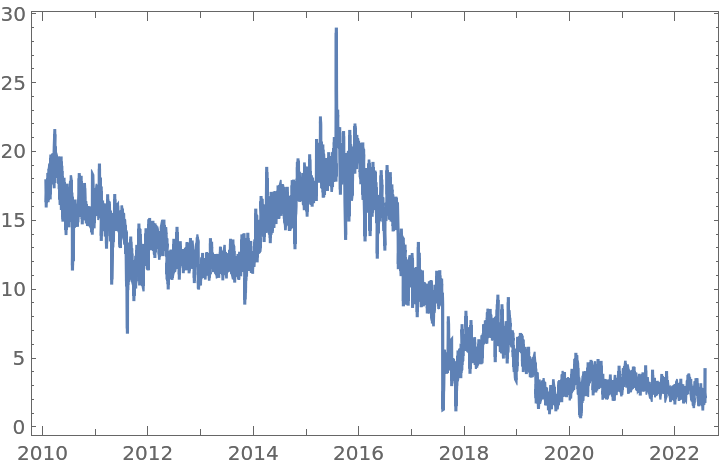In contrast, a fractional difference of the same order 0.3531, but with a smaller tolerance, will generate a time series with fewer data points:

 In:=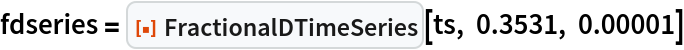Out=In:=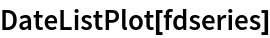Out=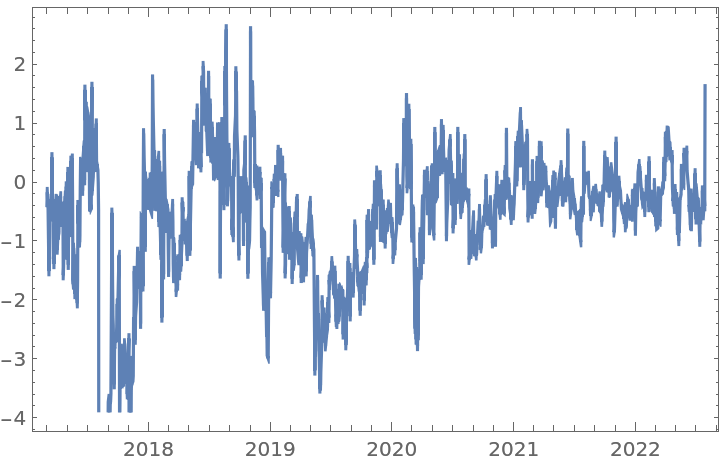### Applications (3)

Simulate an ARIMAProcess with linear trend and apply a fractional difference of order 0.5:

 In:=Out=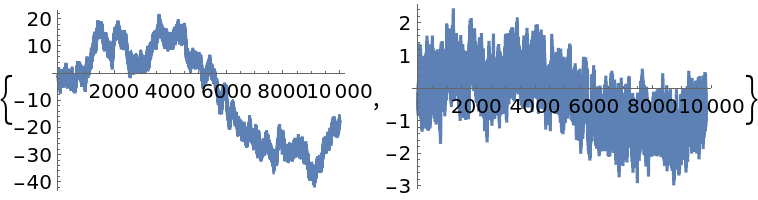Simulate an FractionalBrownianMotionProcess with Hurst index 0.65 and apply a fractional difference of order 0.45 to it:

 In:=Out=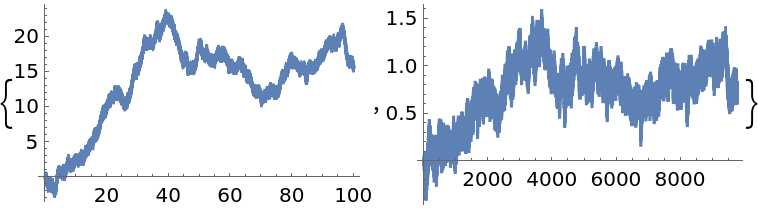Get a time series of the frequencies of the word "Peru" in typical published text, and apply a fractional difference of order 0.35 and a tolerance of 0.01 to it:

 In:=Out=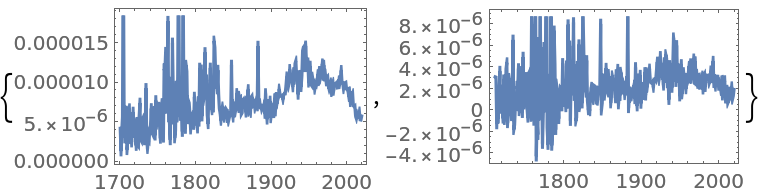### Possible Issues (4)

FractionalDTimeSeries requires the order to be a positive real number. Negative orders (integration) are currently not implemented:

 In:=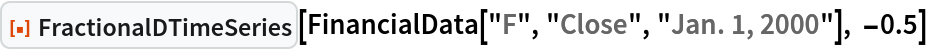Out=FractionalDTimeSeries requires the tolerance to be positive:

 In:=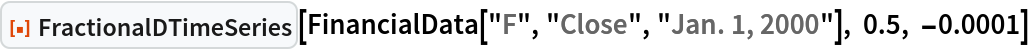Out=If the tolerance is too small, no transformation will be performed:

 In:=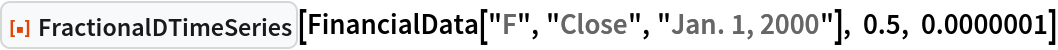Out=If the tolerance is set too high, the transformed time series will be essentially the same as the original one, but in a different scale:

 In:=Out=In:=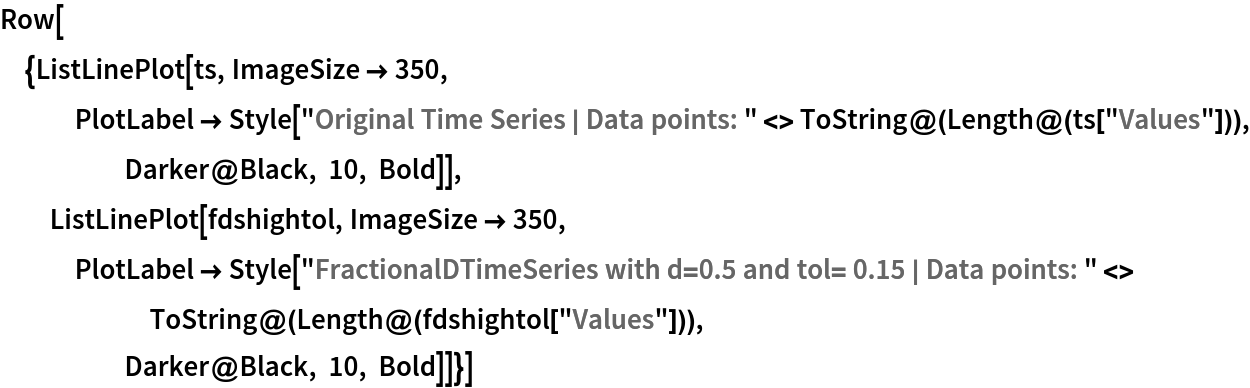Out=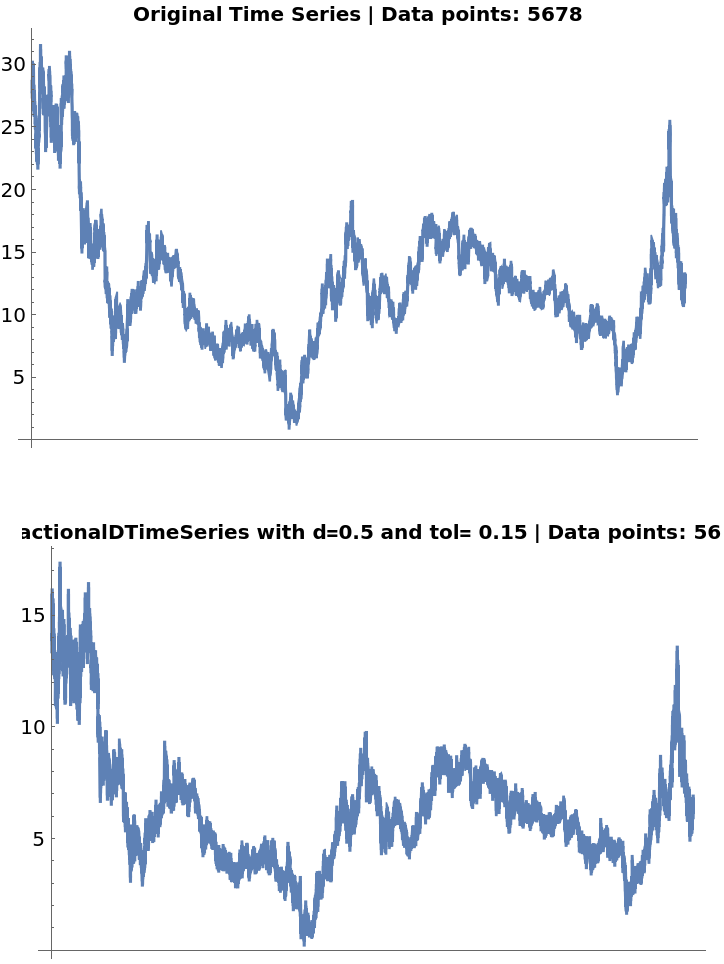### Neat Examples (4)

A logarithmically-transformed time series:

 In:=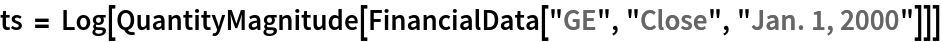Out=In:=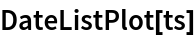Out=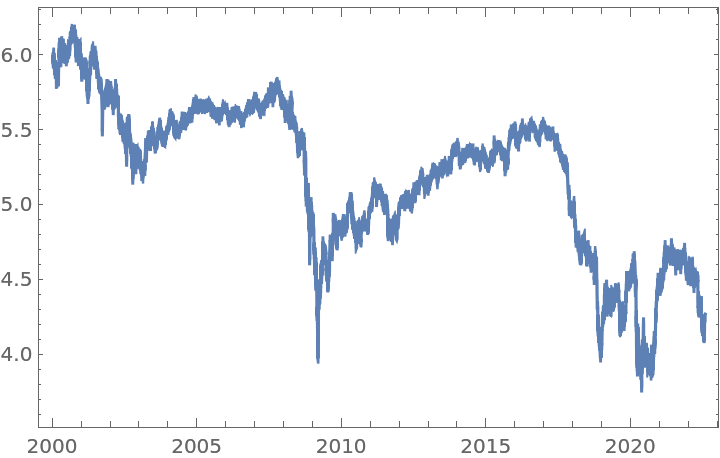Find the relationship between the statistical value of a UnitRootTest and the CorrelationFunction of a transformed time series, by using several orders between 0 and 1:

 In:=Apply a UnitRootTest (red axis) on each differentiated time series to see up from which level of d is possible to reject H0. Also, compute its CorrelationFunction at lag 1 (blue axis) to compare:

 In:=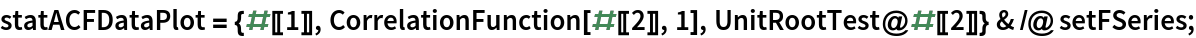Plot the results:

 In:=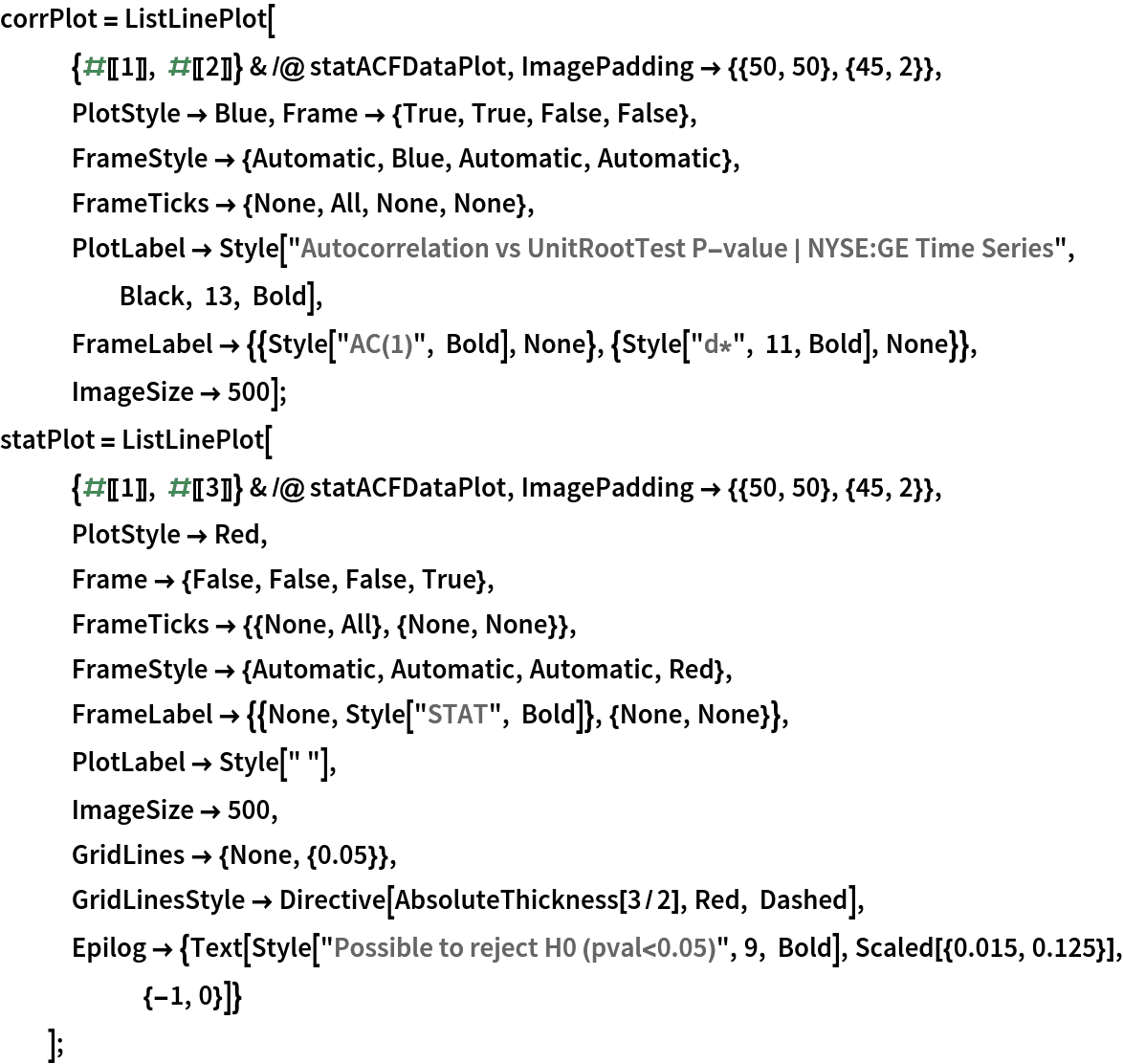In:=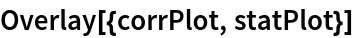Out=